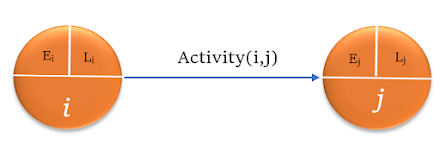# Forward and Backward Pass- Determine Start and Finish Time of an Activity

Forward and backward pass method is employed in construction network scheduling to determine the earliest and latest occurrence time of each event or activity. In forward pass, the calculation starts from the first event or node to the final node. In backward pass, the time is calculated in reverse direction, i.e from the last node or event to the first node.

The start and finish times of the activity are:
1. Earliest Start Time (EST) / Ei
2. Latest Start Time (LST) /Li
3. Earliest Finish Time (EFT) /Ej
4. Latest Finish Time (LFT) /Lj

The forward pass is used to determine the earliest occurrence time ( EST and EFT), while the Backward pass method is used to determine the latest occurrence time (EFT and LFT)An Activity A(i,j) with nodes i and j.  Start and Finish times are Ei, Li, Ej, and Lj

Note: Here i and j are nodes or events. Event times are represented as Ei, Li, Ej and Ej. If we are considering activity, then we represent as EST(i,j), LST (i,j), EFT (i,j), LFT (i,j). Where,
EST (i,j) = Ei;
LST (i,j) = Li;
EFT (i,j) = Ej
LFT (i,j) = Lj;

Whatever way you represent, the below calculation can be applied the same for both. Here the forward pass and backward pass are discussed considering the time occurrence as event times.

Always follow a single type of representation either EST or E while doing problems to avoid confusion.

Given below is a project network, with the activities  A (1,2) - 4 days, B (2,3)- 5 days, C (2,4) - 7 days, D(3,5)- 2 days, E (4,5) -3 days, F(5,6)- 5 days. General representation as Activity Name ( i,j); where i and j are nodes.

### Forward Pass Method to Determine Earliest Occurrence Times of an Activity

In the forward pass method, the calculation moves from the "start" node and ends towards the "finish" node. Here, the start node is 1 and the finish node is 6.

For an Acitivity A (i,j) with duration given as D (i,j);

Ej = Ei + Dij

Node 1 ( i=1)

The start times associated with node 1 are E1 and J1. As it is the start node, E1 is taken zero. Hence,
E1= 0;

Node 2,3 and 4

E2 = E1 + D(1,2) = 0 + 4 = 4;
E3 = E2 + D(2,3) = 4+5 = 9;
E4 = E2 + D (2,4) = 4 + 7 = 11

Node 5

When considering node 5, there are two incoming arrows from node 3 and node 4.

When  arrows go to a single node, then the earliest occurrence time is calculated as follows:
Ei = Maxi [{ Ei + D(i,j)]
Where the maximization is over all node 'i' that precede node 'j'

Hence,
E5 = max[{ E3 + D(3,5)}, {E4 + D(4,5)}] = max{(9+2), (11+3)} = max ( 11, 14) = 1;

Node 6

E6 = E5 + D(5,6) = 14+5 = 19

E6 is the final node whose earliest occurrence time is 19. This is the earliest time for reaching the final node i.e. the minimum time that will be taken to complete the project

The earliest occurrence time of the final node of a network is the minimum time required to complete the project.

### Backward Pass Method to Determine Late Occurrence Times of an Activity

The backward pass method is performed from the last node ( Node 6) of the network and it proceeds in the reverse direction. The late occurrence times are estimated as L6, L5, L4, L3, L2, and L1.

The general formula for determining the late occurrence time is
Li = Lj - D(i,j);

Node 6

This is the end node of the network. The late occurrence time is taken equal to the earliest occurrence time. Therefore,

L6 = E6 = 19;

Node 5,4,3

Here, as per the formula,

L5 = L6 - D(5,6) = 19-5=14
L4=L5 - D(4,5) = 14-3 =11
L3= L5-D(3,5) = 14-2=12

Node 2

There are two outgoing arrows from the node 2 i.e node 3 and 4;

When  arrows go out a single node, then the latest occurrence time is calculated as follows:
Li = MinJ [{ Li -D(i,j)]
Where the maximization is over all node ' J' that precedes node 'i'.

Hence,

L2 = min{ L3-D(2,3), L4 - D(2,4)}] = min[{ 12-5}, {11-7}] = min[7,4] = 4;

Node 1

L1 = L2- D(1,2) = 4 - 4 = 0;

The backward process ends at the first node.

In the network diagram, it is seen that in some of the events or nodes, the E and L values are the same, when the latest project completion time is taken as the earliest project completion time. These events constitute the critical events and the continuous path through them gives the critical path.